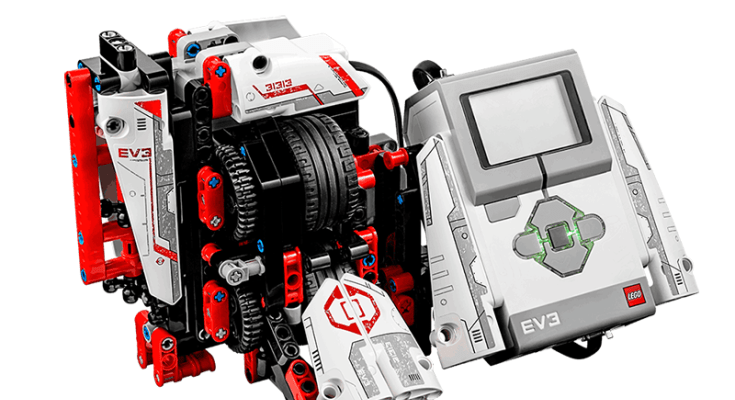# Mr. B3am

Python code and building instructions for the LEGO MINDSTORMS EV3 Official Fan Creations (31313).Image credit: LEGO

This funny-looking robot is ready to organize all your LEGO® Technic beams. Simply insert the beams into the machine, and MR B3AM will detect their color and size.

This program requires LEGO® EV3 MicroPython v2.0 downloadable at https://education.lego.com/en-us/support/mindstorms-ev3/python-for-ev3.

NOTE: Mr. B3am can detect and measure accurately Black and Red Technic beams.

The code for the `MrB3am` class is in `mr_b3am.py` as follows:

``````from pybricks.hubs import EV3Brick
from pybricks.ev3devices import Motor, ColorSensor
from pybricks.media.ev3dev import SoundFile
from pybricks.parameters import Color, Direction, Port, Stop
from pybricks.tools import wait

class MrB3am:
def __init__(
self,
gear_motor_port: Port = Port.A,
color_sensor_port: Port = Port.S3):
self.ev3_brick = EV3Brick()

self.gear_motor = Motor(port=gear_motor_port,
positive_direction=Direction.CLOCKWISE)

self.color_sensor = ColorSensor(port=color_sensor_port)

def header_text(self):
self.ev3_brick.screen.clear()
self.ev3_brick.screen.draw_text(
x=0, y=0,
text='MR. B3AM',
text_color=Color.BLACK,
background_color=None)

def insert_b3am(self):
"""
This waits for a B3am to be inserted.
A B3am is detected when the ambient light level is
above or equal to the value 3.
When a B3am is inserted the motor stops
and the EV3 Brick says "Thank you".
"""
self.header_text()

self.ev3_brick.screen.draw_text(
x=0, y=15,
text='Insert B3am!',
text_color=Color.BLACK,
background_color=None)

self.gear_motor.run(speed=-150)

while self.color_sensor.reflection() < 3:
pass

self.gear_motor.hold()

self.ev3_brick.speaker.play_file(SoundFile.THANK_YOU)

def measure_b3am(self):
"""
This measures the length of the B3am,
by first resetting the motor counter and then moving the B3am
until the other end is found.
This is detected when the ambient light level is below the value 1.
Note that the length measured is the number of degrees
that the wheels has turned.
This value will later be converted to the actual B3am length.
After having found the length of the B3am, the length is saved
in a variable, named "current_b3am_length_in_degrees".
"""
self.header_text()

self.ev3_brick.screen.draw_text(
x=0, y=15,
text='Measuring B3am...',
text_color=Color.BLACK,
background_color=None)

self.gear_motor.reset_angle(angle=0)

self.gear_motor.run(speed=-150)

while self.color_sensor.reflection() > 1:
wait(10)

self.gear_motor.hold()

self.current_b3am_length_in_degrees = abs(self.gear_motor.angle())

def detect_color(self):
"""
Afterwards the B3am is moved half way thorugh the machine
so its color can be measured.
When the color is found it is saved in a variable,
named "current_b3am_color_code" and the EV3 Brick says "Detected".
Note the saved value is the color ID and this will later be converted
to the actual color name.
"""
self.header_text()

self.ev3_brick.screen.draw_text(
x=0, y=15,
text='Detecting Color...',
text_color=Color.BLACK,
background_color=None)

self.gear_motor.run_angle(
speed=150,
rotation_angle=self.current_b3am_length_in_degrees / 2,
then=Stop.HOLD,
wait=True)

self.current_b3am_color_code = self.color_sensor.color()

self.ev3_brick.speaker.play_file(SoundFile.DETECTED)

def eject_b3am(self):
"""
After the color is found, the EV3 calculates the number of degrees
required to move the wheels,
such that the B3am is ejected from the machine.
"""
self.header_text()

self.ev3_brick.screen.draw_text(
x=0, y=15,
text='Ejecting B3am...',
text_color=Color.BLACK,
background_color=None)

self.gear_motor.run_angle(
speed=150,
rotation_angle=self.current_b3am_length_in_degrees / 2 + 700,
then=Stop.HOLD,
wait=True)

def process_b3am(self):
self.insert_b3am()

self.measure_b3am()

self.detect_color()

self.eject_b3am()

def report_result(self, debug=False):
"""
Report the result of the measurement.
The switch to the right has a case for each color
the Color Sensor is able to detect.
MR-B3AM converts from the number of rotation degrees to B3am lengths.
"""
self.header_text()

if self.current_b3am_color_code == Color.BLACK:
self.current_b3am_color = 'black'

if 400 <= self.current_b3am_length_in_degrees <= 600:
self.current_b3am_length = 5

elif 601 <= self.current_b3am_length_in_degrees <= 800:
self.current_b3am_length = 7

elif 801 <= self.current_b3am_length_in_degrees <= 1000:
self.current_b3am_length = 9

elif 1001 <= self.current_b3am_length_in_degrees <= 1300:
self.current_b3am_length = 11

elif 1301 <= self.current_b3am_length_in_degrees <= 1500:
self.current_b3am_length = 13

elif 1501 <= self.current_b3am_length_in_degrees <= 1700:
self.current_b3am_length = 15

elif self.current_b3am_color_code == Color.RED:
self.current_b3am_color = 'red'

if 400 <= self.current_b3am_length_in_degrees <= 800:
self.current_b3am_length = 5

elif 801 <= self.current_b3am_length_in_degrees <= 1050:
self.current_b3am_length = 7

elif 1051 <= self.current_b3am_length_in_degrees <= 1300:
self.current_b3am_length = 9

elif 1301 <= self.current_b3am_length_in_degrees <= 1500:
self.current_b3am_length = 11

elif 1501 <= self.current_b3am_length_in_degrees <= 1700:
self.current_b3am_length = 13

elif 1701 <= self.current_b3am_length_in_degrees <= 1900:
self.current_b3am_length = 15

else:
self.current_b3am_color = 'UNKNOWN'
self.current_b3am_length = 'UNKNOWN'

self.ev3_brick.screen.draw_text(
x=0, y=15,
text='Color: {}'.format(self.current_b3am_color.upper()),
text_color=Color.BLACK,
background_color=None)

self.ev3_brick.screen.draw_text(
x=0, y=30,
text='Length: {}'.format(self.current_b3am_length),
text_color=Color.BLACK,
background_color=None)

if debug:
self.ev3_brick.screen.draw_text(
x=0, y=60,
text='{}'.format(self.current_b3am_color_code),
text_color=Color.BLACK,
background_color=None)

self.ev3_brick.screen.draw_text(
x=0, y=75,
text='Degrees: {:,}'.format(
self.current_b3am_length_in_degrees),
text_color=Color.BLACK,
background_color=None)

self.ev3_brick.speaker.say(
text='{color} {length}{n_degrees}'.format(
color=self.current_b3am_color,
length=self.current_b3am_length,
n_degrees=' ({} Degrees)'.format(
self.current_b3am_length_in_degrees)
if debug
else ''))

``````

The code for the main program is in `main.py` as follows:

``````#!/usr/bin/env pybricks-micropython

from pybricks.parameters import Button, Color
from pybricks.tools import wait

from mr_b3am import MrB3am

mr_b3am = MrB3am()

while True:
mr_b3am.process_b3am()

mr_b3am.report_result(debug=True)

mr_b3am.ev3_brick.screen.draw_text(
x=0, y=105,
text='Press Enter...',
text_color=Color.BLACK,
background_color=None)

while Button.CENTER not in mr_b3am.ev3_brick.buttons.pressed():
wait(10)

``````

This project was submitted by The Lương-Phạm Family.